• 微积分探讨的三大思想：

1. Integrals 积分 $\int^R_0$

2. Derivatives 求导 $\frac{d}{dx}$

3. Fact that they are opposite 它们的关系

• 当你使用 $dt$ 时，你只是想表示 $dt\rightarrow 0$，它既不是「不穷小」，也不是 $0$。

• $a^t$ 可以表示为 $e^{ct}$，其中$c=\ln a$。为什么选用 $e$ 而不是其他底？

• $a^{kt} = k \times \ln a\times a^{kt}$ （多了 $\ln a$）

• $e^{kt} = k \times e^{kt}$ （$k$ 即为变化率）

符合自然中的许多现象，变化率总是和变化量成正比：

• 人口增长

• 温度变化

• 金钱投资

• 位移 $s(t)$ 的导数：

• $\frac{ds}{dt}$ Velocity

• $\frac{d^2s}{dt^2}$ Acceleration

• $\frac{d^3s}{dt^3}$ Jerk（急动度）

• Chain Rule 的直观理解：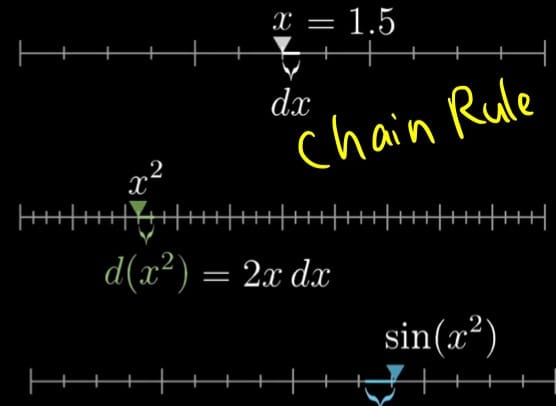• 泰勒展开式每一项的系数可以看作是一种控制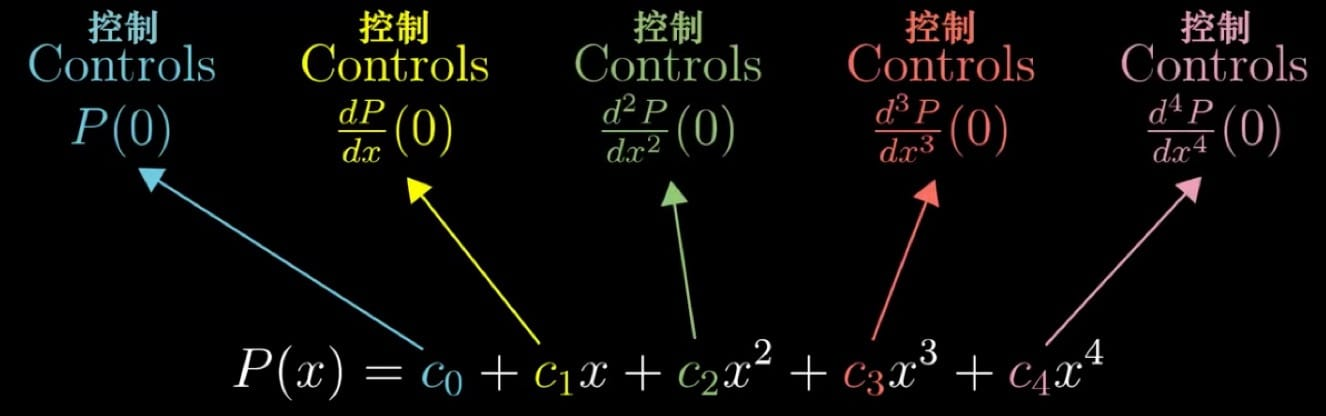• 夹逼定理（Squeeze Theorem）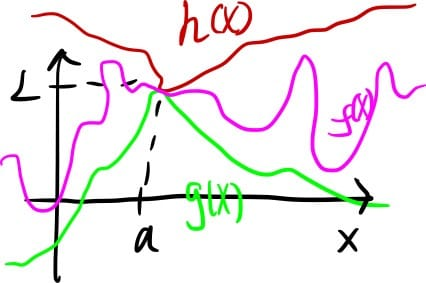• 连续性

• 定义：设函数 $y=f(x)$ 在点 $x_0$ 的某一领域内有定义，如果$\lim\limits_{\Delta x \rightarrow 0}\Delta y = \lim\limits_{\Delta x \rightarrow 0}[f(x_0 + \Delta x) - f(x_0)]=0，$

那么称 $y=f(x)$ 在点 $x_0$ 上连续。

• 函数极限的性质

• 唯一性

• 有界性：如果 $\lim\limits_{x \rightarrow x_0}f(x)=A$，则存在常数 $M > 0$ 和 $\delta > 0$，使得当 $0 < |x - x_0| < \delta$ 时，有 $|f(x)|\leq M$

• 局部保号性

• 无穷小（Infinitesimal or Infinitely Small）

• 定义：如果 $f(x)$ 当 $x\rightarrow x_0$（或 $x\rightarrow \infty$）时的极限为 $0$，那么称 $f(x)$ 为当 $x\rightarrow x_0$（或 $x\rightarrow \infty$）时的无穷小。不要把它和很小的数混为一谈！

• 无穷大（也不是数，只是极限趋于无穷大）

• 无穷小的比较 → 反映了不同的无穷小趋近的快慢

• $\lim \frac{\beta}{\alpha} = 0$，$\beta$ 比 $\alpha$ 高阶的无穷小，记为 $\beta=o(\alpha)$

• $\lim \frac{\beta}{\alpha} = \infty$，$\beta$ 比 $\alpha$ 低阶的无穷小

• $\lim \frac{\beta}{\alpha} = c \neq 0$，同阶无穷小

• $\lim \frac{\beta}{\alpha^k} = c \neq 0$，$\beta$ 是 $\alpha$ 的 $k$ 阶无穷小

• $\lim \frac{\beta}{\alpha}=1$，等价无穷小，记为 $\alpha \sim \beta$

• 定理 1：$\beta$ 与 $\alpha$ 是等价无穷小的充要条件为 $\beta=\alpha+o(\alpha)$。

当 $x \rightarrow 0$ 时，$\sin(x) \sim x、\tan(x)\sim x、\arcsin(x)\sim x、1-\cos(x)\sim\frac{1}{2}x^2$

• 定理 2：设 $\alpha \sim \alpha’，\beta \sim \beta’$，且 $\frac{\lim \beta’}{\lim \alpha’}$ 存在，则 $\lim \frac{\beta}{\alpha} = \lim \frac{\beta’}{\alpha’}$。

• 不连续点或间断点

• 满足三种情况之一：

• 在 $x=x_0$ 没有定义

• 在 $x=x_0$ 有定义，但在 $\lim\limits_{x\rightarrow x_0}f(x)$ 不存在

• 在 $x=x_0$ 有定义，但 $\lim\limits_{x\rightarrow x_0}f(x) \neq f(x_0)$

• 一类间断点（左右极限存在）

• 可去间断点（极限相等）

• 跳跃间断点（极限不相等）

• 二类间断点（左右极限不存在）

• 无穷间断点

• 振荡间断点

• 区分「微分 Differential」和「导数 Derivative」

• 微分的定义：设 $y=f(x)$ 在某区间内有定义，增量 $\Delta y = f(x_0 + \Delta x)-f(x_0)$ 可以表示为 $\Delta y = A\Delta x + o(\Delta x)$，其中 $A$ 是不依赖于 $\Delta x$ 的常数「$A=f’(x)$」，那么称 $f(x)$ 在 $x =x_0$ 上是可微的，则 $A\Delta x$ 也叫作改点上的微分，记作 $dy$，即 $dy = A\Delta x$。

• 几何意义：切线的一元线性方程。局部切线段近似代替曲线段，即非线性函数的局部化。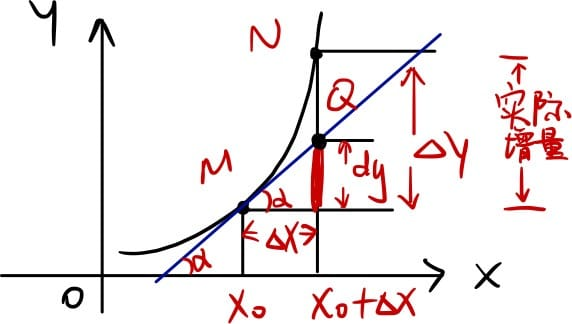• 导数是函数到函数的映射，而微分是求另一个与导函数有关的函数。对于一元函数可能看不出什么区别，但对于二元函数，没法同时对 $x$ 和 $y$ 求导，但可以同时对它们求微分。比如在工程中常用：

如 $\sqrt[n]{1+x}\approx 1+\frac{1}{n}x$、$e^x \approx x$。

• 全微分：$\Delta z =f(x+\Delta x, y+\Delta y) - f(x,y) = \frac{\partial z}{\partial x}\Delta x + \frac{\partial z}{\partial y}\Delta y + o(\sqrt{(\Delta x)^2+(\Delta y)^2})$

• 微分中值定理

• 条件：
• 在 $[a, b]$ 上连续
• 在 $(a, b)$ 上可导
• 不同❓
1. 罗尔定理：至少有一点 $f’(\xi)=0$

2. 拉格朗日中值定理：$\frac{f(b)-f(a)}{b-a}=f’(\xi)$

3. 柯西中值定理：$\frac{f(b)-f(a)}{F(b)-F(a)}=\frac{f’(\xi)}{F’(\xi)}$，当 \left\{\begin{aligned} X=F(x)\\Y=f(x) \end{aligned} \right.(a \leq x \leq b)

• 泰勒中值定理

• 如果函数 $f(x)$ 在含有 $x_0$ 的某个区间 $(a,b)$ 内具有直到 $(n+1)$ 阶的导数，则对任一 $x\in(a,b)$ 有：

其中 $R_n(x) = \frac{f^{(n+1)}(x_0)}{(n+1)!}(x-x_0)^{n+1}，(x_0 \leq \xi \leq x)$，为拉格朗日型余项；而 $R_n(x) = o[(x-x_0)^n]$ 为佩亚诺型余项。

• 函数凹 / 凸

• $f(\frac{x_1+x_2}{2}) < \frac{f(x_1)+f(x_2)}{2} \Leftrightarrow f’’(x) > 0$ （凹，极小值）

• $f(\frac{x_1+x_2}{2}) > \frac{f(x_1)+f(x_2)}{2} \Leftrightarrow f’’(x) < 0$ （凸，极大值）

• $f’’(x) = 0$ 对应的点称为拐点（Inflection Point），凹凸都有可能。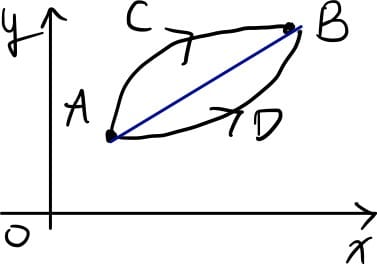• 换元积分法

• 第一类：设 $f(u)$ 具有原函数，$u=\varphi(x)$ 可导，则有换元公式$\int f[\varphi(x)]\varphi'(x)dx = [\int f(u)du]_{u=\varphi(x)}$

• 第二类：$\int f(x)dx = \int f[\psi(t)]\psi'(t)dt$

• 定积分中值定理

• 如果函数 $f(x)$ 在积分区间 $[a,b]$ 上连续，则在 $[a,b]$ 上至少存在一个点 $\xi$，使下式成立：$\int^b_a f(x)dx=f(\xi)(b-a)，(a \leq \xi \leq b)$

$f(\xi)$ 是平均值。

• 微分方程

• 定义：表示未知函数（$y$）、未知函数的导数（$\frac{dy}{dx}$）与自变量（$x$）之间的关系的方程，如 $\frac{dy}{dx}=2xy$、$x^3y’’’=3x^2$。

• 求解：如上面方程通过变量分离后得 $\frac{dy}{y}=2xdx$，再对两端积分得 $y=\pm e^{x^2+C_1}$。

• 平面及其方程

• 三元方程：$F(x,y,z)=0$

• 平面点法式方程：$\vec{n}\cdot\overrightarrow{M_0 M} = 0 \Leftrightarrow A(x-x_0)+B(y-y_0)+C(z-z_0)=0$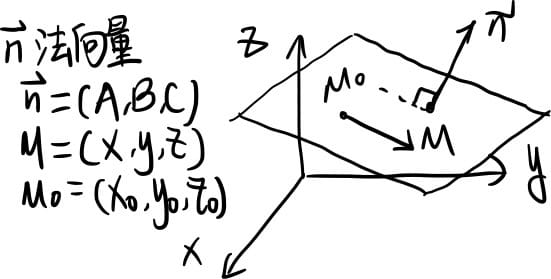• 一般方程：$Ax+By+Cz+D=0，\vec{n}=(A,B,C)$

• 截距式方程：$\frac{x}{a}+\frac{y}{b}+\frac{z}{c}=1$

• 空间直线及其方程• 一般方程：\left\{\begin{aligned}A_1x+B_1y+C_1z+D_1=0\\A_2x+B_2y+C_2z+D_2=0\end{aligned}\right.

• 对称式方程（点向式方程）：$\frac{x-x_0}{m}=\frac{y-y_0}{n}=\frac{z-z_0}{p}$

• 参数方程：\left\{\begin{aligned}x&=x_0+mt\\y&=y_0+nt\\z&=z_0+pt\end{aligned}\right.

• 空间曲线及其方程

• 一般方程：\left\{\begin{aligned}F(x,y,z)=0\\G(x,y,z)=0\end{aligned}\right.

• 参数方程：\left\{\begin{aligned}x=x(t)\\y=y(t)\\z=z(t)\end{aligned}\right.

0%